# Selina Solutions Concise Mathematics Class 6 Chapter 6: Negative Numbers And Integers

Selina Solutions help students in improving their academic performance. These solutions are 100% accurate and reliable resources to obtain high marks in exams. The in-depth practice of Selina Solutions Concise Mathematics enhances students’ skills and confidence to face examinations fearlessly. By practicing these solutions, students who find difficulty while solving textbook questions become more proficient in Mathematics and solve with ease. This also strengthens conceptual knowledge among students.

An integer is defined as a number that has no fractional part and no digits after the decimal point. It can be positive, negative or zero. Selina Solutions are helpful in clearing students’ doubts instantly. Subject experts have prepared the solutions for students, based on the current ICSE exam, in order to boost their exam preparation. Students are advised to download the PDF of Selina Solutions Concise Mathematics Class 6 Chapter 6 Negative Numbers And Integers, from the links given here.

## Selina Solutions Concise Mathematics Class 6 Chapter 6: Negative Numbers And Integers Download PDF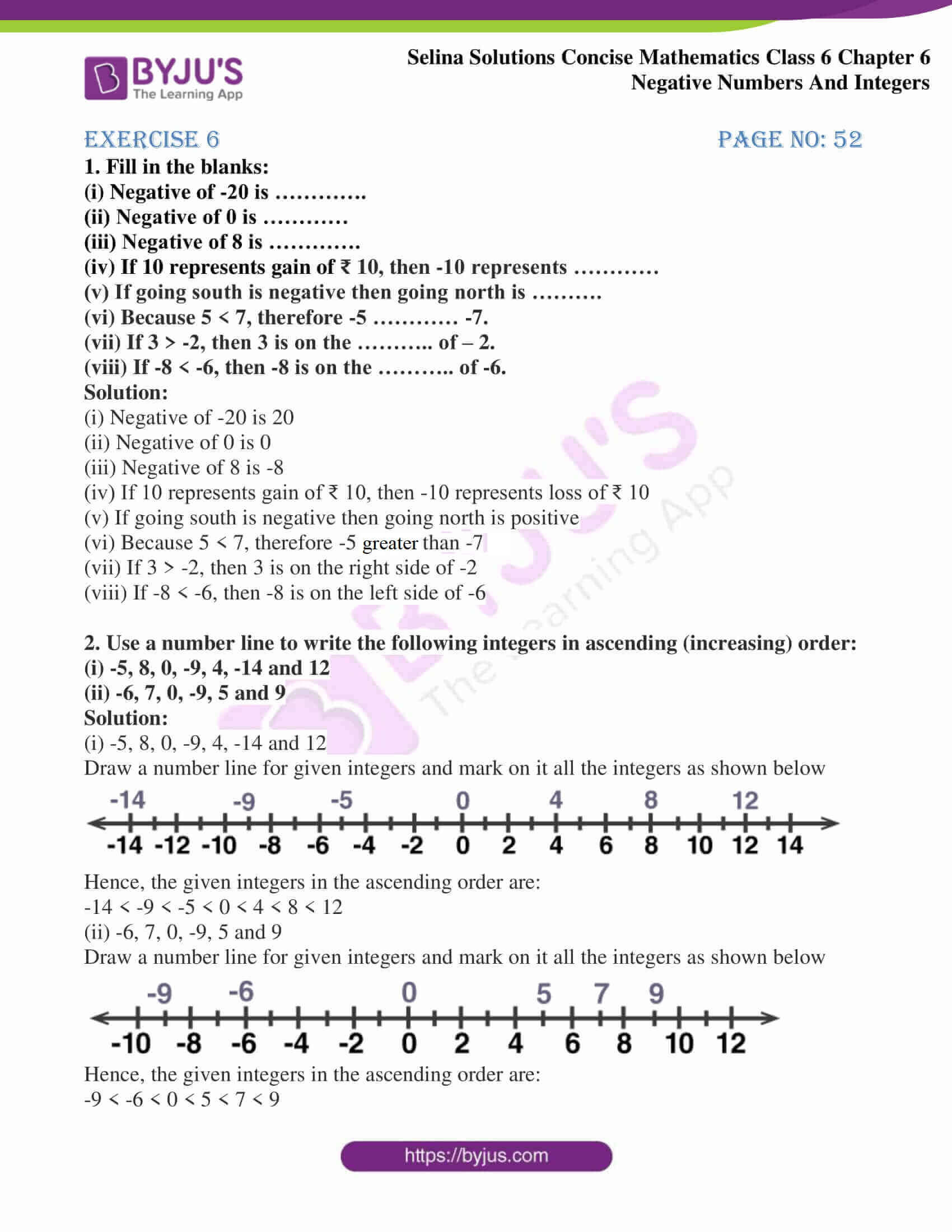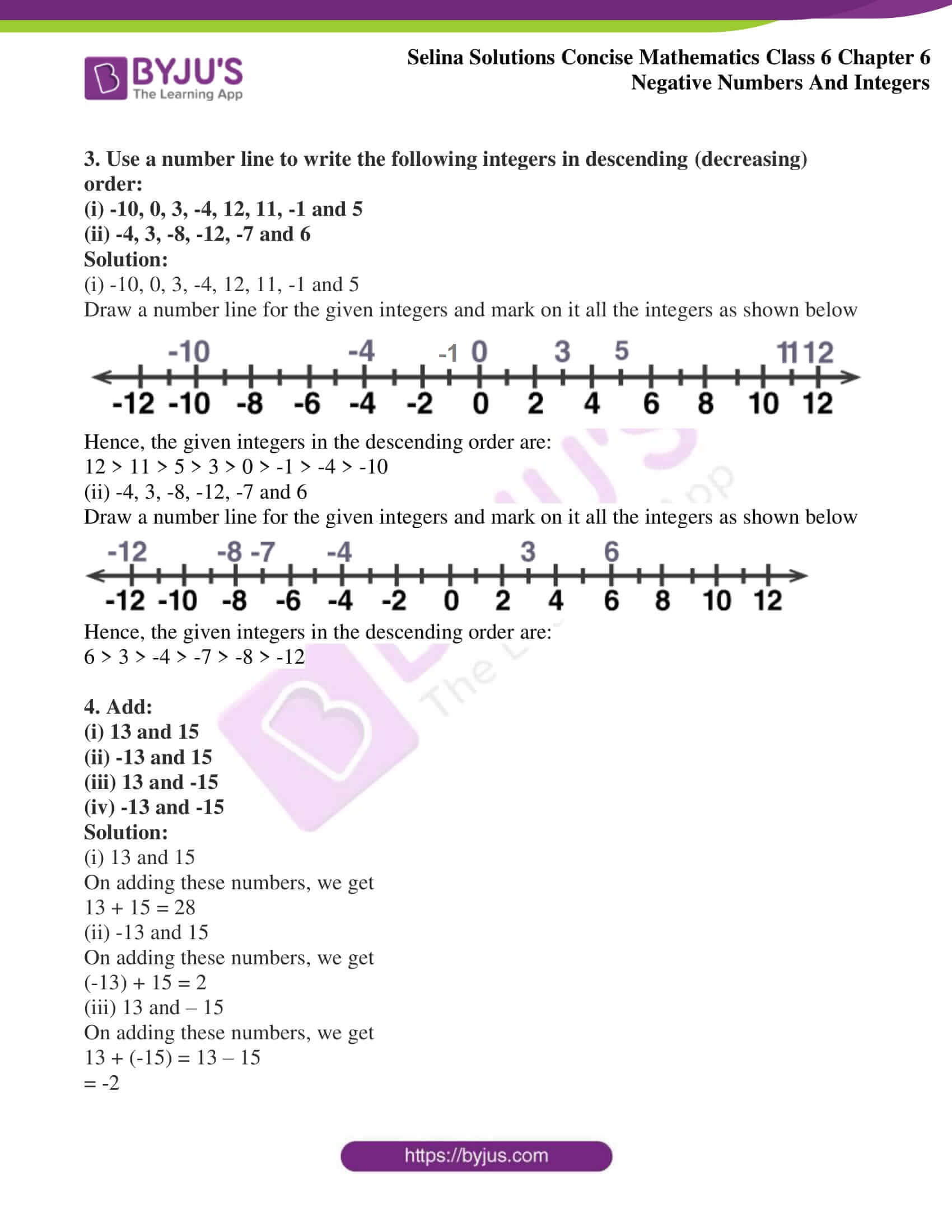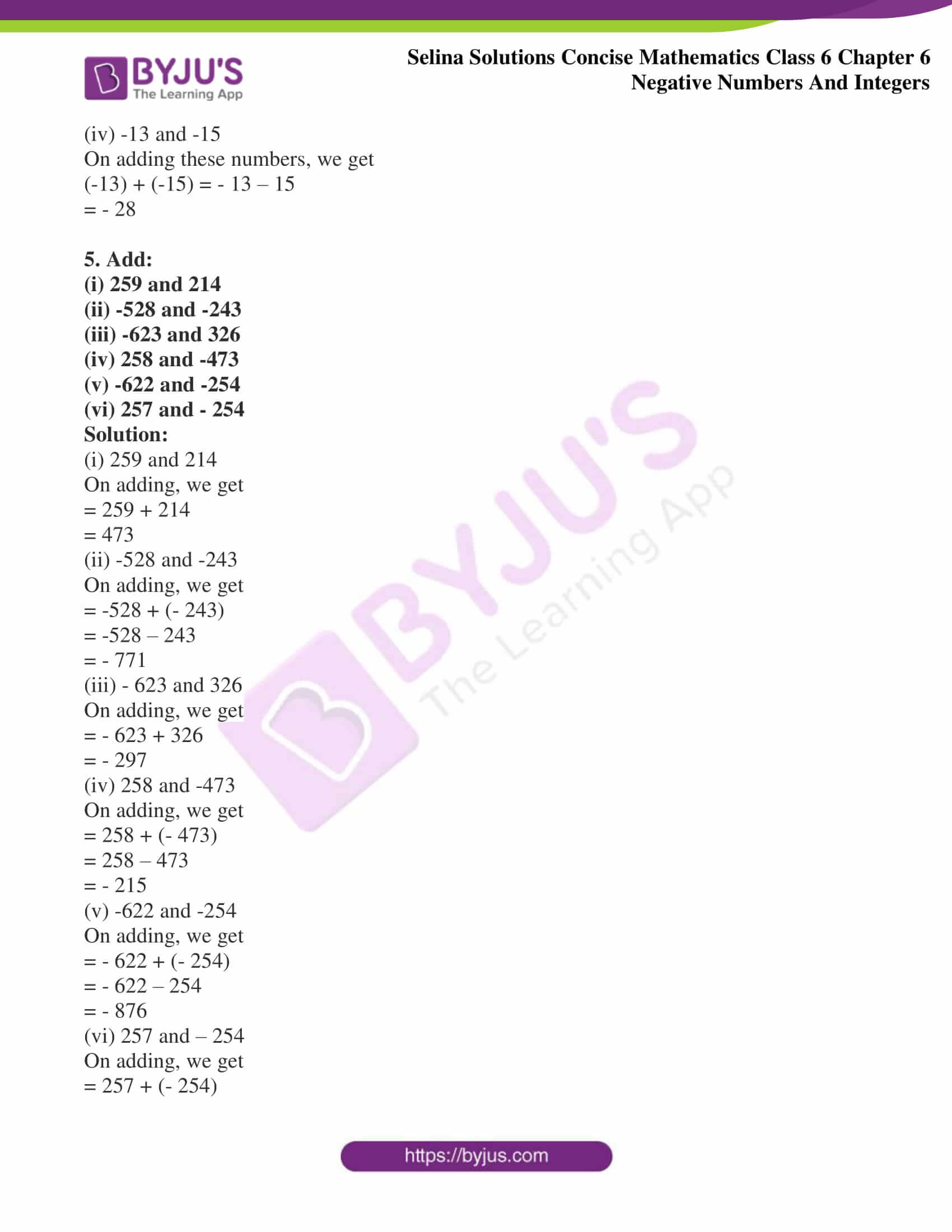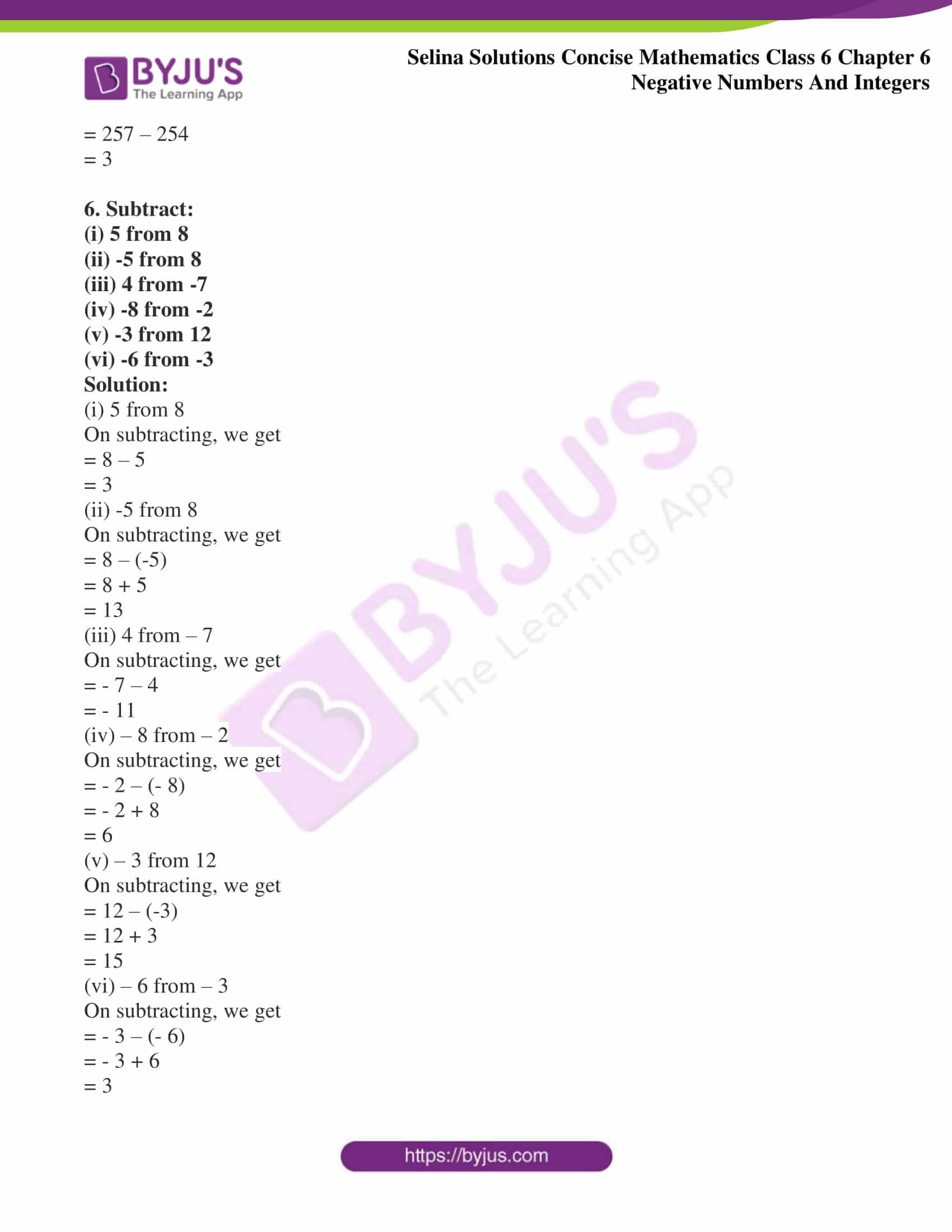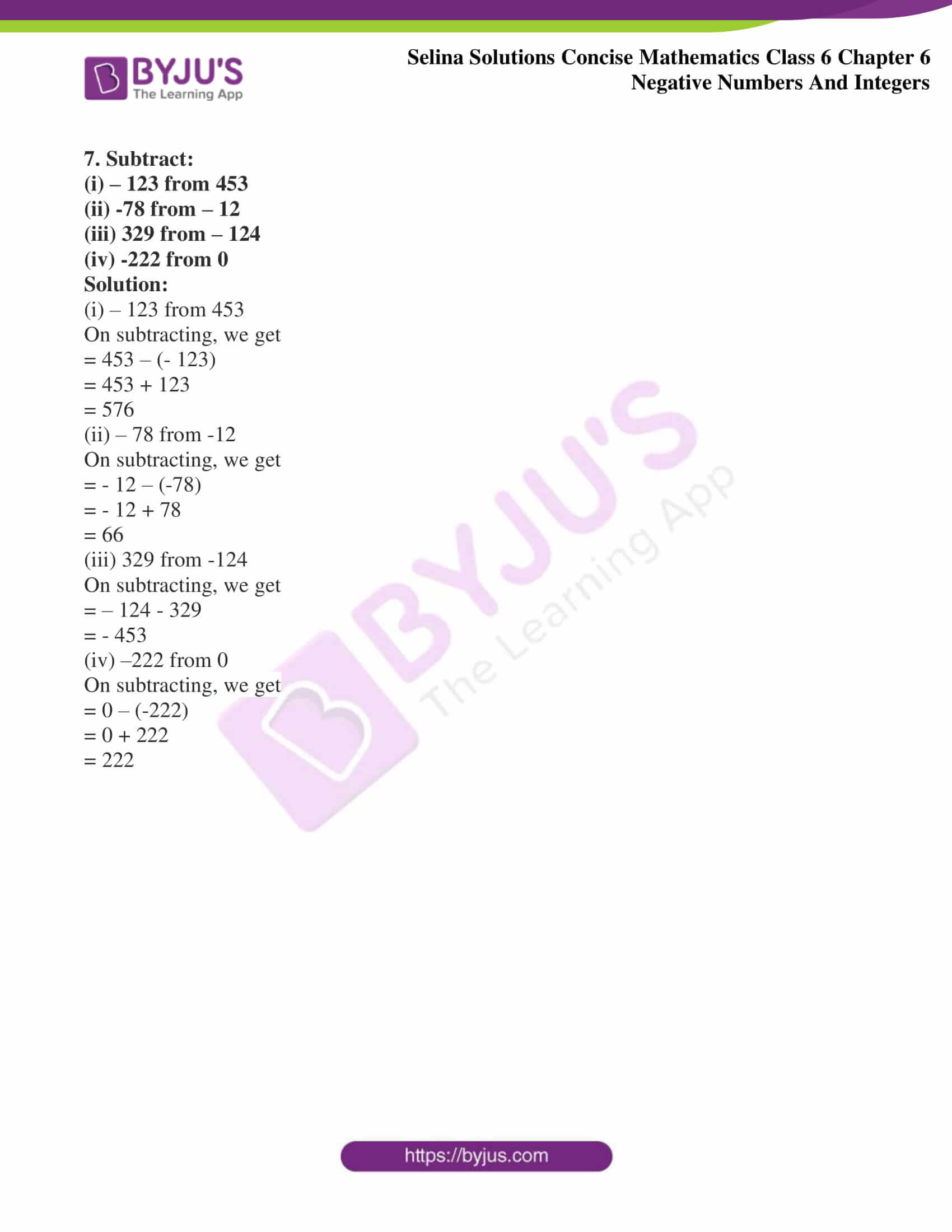## Access Selina Solutions Concise Mathematics Class 6 Chapter 6: Negative Numbers And Integers

Exercise 6 page no: 52

1. Fill in the blanks:

(i) Negative of -20 is ………….

(ii) Negative of 0 is …………

(iii) Negative of 8 is ………….

(iv) If 10 represents gain of ₹ 10, then -10 represents …………

(v) If going south is negative then going north is ……….

(vi) Because 5 < 7, therefore -5 ………… -7.

(vii) If 3 > -2, then 3 is on the ……….. of – 2.

(viii) If -8 < -6, then -8 is on the ……….. of -6.

Solution:

(i) Negative of -20 is 20

(ii) Negative of 0 is 0

(iii) Negative of 8 is -8

(iv) If 10 represents gain of ₹ 10, then -10 represents loss of ₹ 10

(v) If going south is negative then going north is positive

(vi) Because 5 < 7, therefore -5 greater than -7

(vii) If 3 > -2, then 3 is on the right side of -2

(viii) If -8 < -6, then -8 is on the left side of -6

2. Use a number line to write the following integers in ascending (increasing) order:

(i) -5, 8, 0, -9, 4, -14 and 12

(ii) -6, 7, 0, -9, 5 and 9

Solution:

(i) -5, 8, 0, -9, 4, -14 and 12

Draw a number line for given integers and mark on it all the integers as shown below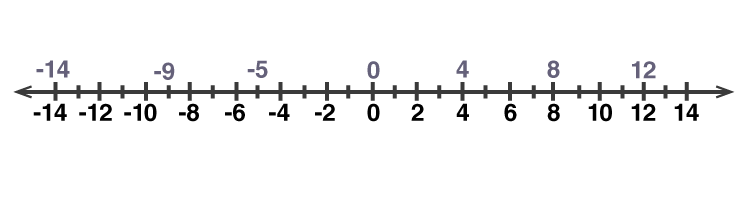Hence, the given integers in the ascending order are:

-14 < -9 < -5 < 0 < 4 < 8 < 12

(ii) -6, 7, 0, -9, 5 and 9

Draw a number line for given integers and mark on it all the integers as shown below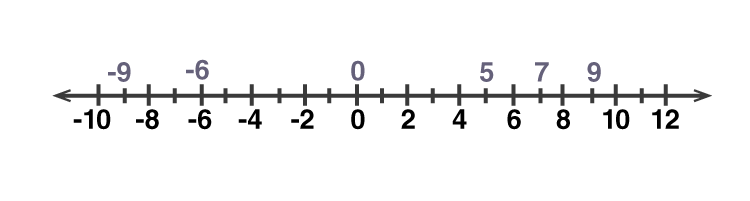Hence, the given integers in the ascending order are:

-9 < -6 < 0 < 5 < 7 < 9

3. Use a number line to write the following integers in descending (decreasing) order:

(i) -10, 0, 3, -4, 12, 11, -1 and 5

(ii) -4, 3, -8, -12, -7 and 6

Solution:

(i) -10, 0, 3, -4, 12, 11, -1 and 5

Draw a number line for the given integers and mark on it all the integers as shown below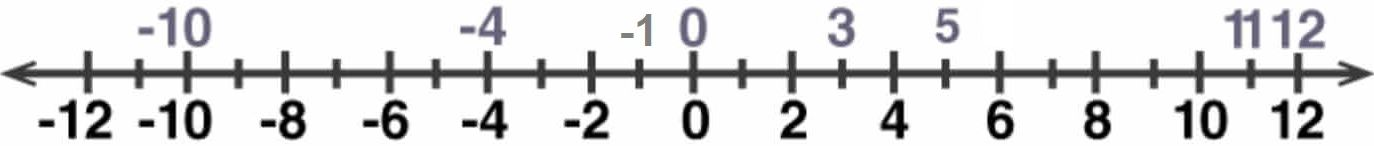Hence, the given integers in the descending order are:

12 > 11 > 5 > 3 > 0 > -1 > -4 > -10

(ii) -4, 3, -8, -12, -7 and 6

Draw a number line for the given integers and mark on it all the integers as shown below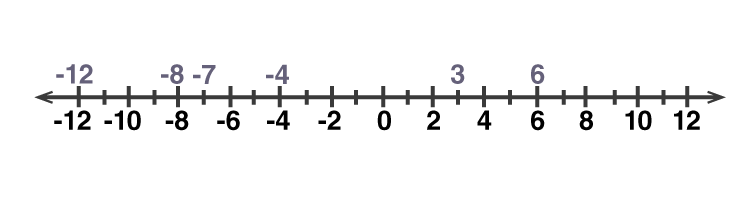Hence, the given integers in the descending order are:

6 > 3 > -4 > -7 > -8 > -12

(i) 13 and 15

(ii) -13 and 15

(iii) 13 and -15

(iv) -13 and -15

Solution:

(i) 13 and 15

On adding these numbers, we get

13 + 15 = 28

(ii) -13 and 15

On adding these numbers, we get

(-13) + 15 = 2

(iii) 13 and – 15

On adding these numbers, we get

13 + (-15) = 13 – 15

= -2

(iv) -13 and -15

On adding these numbers, we get

(-13) + (-15) = – 13 – 15

= – 28

(i) 259 and 214

(ii) -528 and -243

(iii) -623 and 326

(iv) 258 and -473

(v) -622 and -254

(vi) 257 and – 254

Solution:

(i) 259 and 214

= 259 + 214

= 473

(ii) -528 and -243

= -528 + (- 243)

= -528 – 243

= – 771

(iii) – 623 and 326

= – 623 + 326

= – 297

(iv) 258 and -473

= 258 + (- 473)

= 258 – 473

= – 215

(v) -622 and -254

= – 622 + (- 254)

= – 622 – 254

= – 876

(vi) 257 and – 254

= 257 + (- 254)

= 257 – 254

= 3

6. Subtract:

(i) 5 from 8

(ii) -5 from 8

(iii) 4 from -7

(iv) -8 from -2

(v) -3 from 12

(vi) -6 from -3

Solution:

(i) 5 from 8

On subtracting, we get

= 8 – 5

= 3

(ii) -5 from 8

On subtracting, we get

= 8 – (-5)

= 8 + 5

= 13

(iii) 4 from – 7

On subtracting, we get

= – 7 – 4

= – 11

(iv) – 8 from – 2

On subtracting, we get

= – 2 – (- 8)

= – 2 + 8

= 6

(v) – 3 from 12

On subtracting, we get

= 12 – (-3)

= 12 + 3

= 15

(vi) – 6 from – 3

On subtracting, we get

= – 3 – (- 6)

= – 3 + 6

= 3

7. Subtract:

(i) – 123 from 453

(ii) -78 from – 12

(iii) 329 from – 124

(iv) -222 from 0

Solution:

(i) – 123 from 453

On subtracting, we get

= 453 – (- 123)

= 453 + 123

= 576

(ii) – 78 from -12

On subtracting, we get

= – 12 – (-78)

= – 12 + 78

= 66

(iii) 329 from -124

On subtracting, we get

= – 124 – 329

= – 453

(iv) –222 from 0

On subtracting, we get

= 0 – (-222)

= 0 + 222

= 222